# Image operations with NumPy

Martin McBride, 2021-03-14
Tags image processing crop pad fill
Categories numpy pillowIn this section we will see how to use NumPy to perform some basic imaging operations. For more information on NumPy and images, see the maon article. We will look at these operations:

• Crop
• Flip

Although these operations can be performed using an imaging library such as Pillow, there are advantages to using NumPy, especially if the image data is already in NumPy format. it can be a little faster in some cases, but also because you are performing the calculations in your own code it can be a lot more flexible. And it also helps you to understand what is going on under the hood.

We will use this 600 by 400 pixel image as our example in this section:## Cropping images

Cropping an image changes its size by removing pixels from its edges. Here is an example:This image is 300 pixels square, cropped from the centre of the original image.

Here is the code to crop the image:

```import numpy as np
from PIL import Image

img_in = Image.open('boat.jpg')
array = np.array(img_in)

cropped_array = array[50:350, 150:450, :]

img_out = Image.fromarray(cropped_array)
img_out.save('cropped-boat.jpg')
```

First we read the in original image, boat.jpg, using Pillow, and convert it to a NumPy array called `array`. This is the same as we saw in the main article:

```img_in = Image.open('boat.jpg')
array = np.array(img_in)
```

This diagram shows the original image (the outer green rectangle, 600x400 pixels), and the cropped area (the inner blue rectangle, 300x300 pixels). The cropped area starts 150 pixel in from the left of the original image, an 50 pixels down from the top. This places it at the exact centre, although of course you can position it wherever you wish.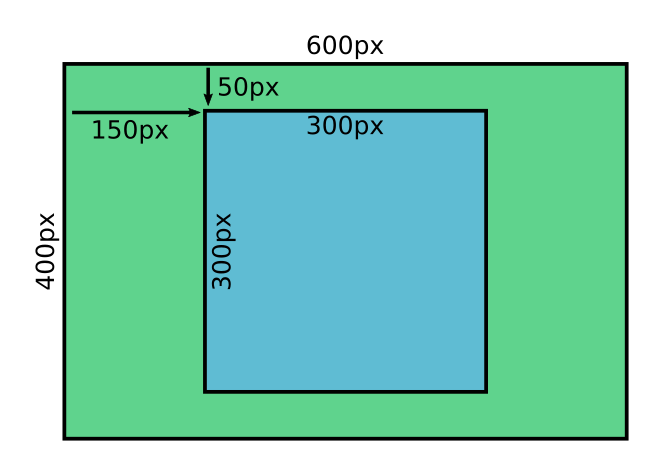To crop the image we simply take a slice:

```cropped_array = array[50:350, 150:450, :]
```

Remember that the first coordinate, represents the row of the NumPy array, which corresponds to the y dimension of the image. We crop from row 50 up to but not including row 350, which gives 300 rows.

The second coordinate represents the colum of the NumPy array, which corresponds to the x dimension of the image. We crop from column 150 up to but not including column 450, which agains gives 300 columns.

The third dimension, which has a length of 3, represents the red, green and blue components of the pixel. We slice the whole of this dimension, because of course we want to copy al three colour planes.

Note that slicing the array gives us a view of the original `array`. `cropped_img` shares the same data as `array`, so if we were to modify one it would also modify the other. We should make a copy of the array if we intended to change it, but in this case we are simply saving `cropped_img` to file without modifying it so we don't need to worry.

Here is the code that saves out cropped image to file, again as we did in the main article.

```img_out = Image.fromarray(cropped_array)
img_out.save('cropped-boat.jpg')
```

This code can be found as cropped-image.py on github.

Padding is (sort of) the opposite of cropping. We make the image bigger by adding a borders, like this: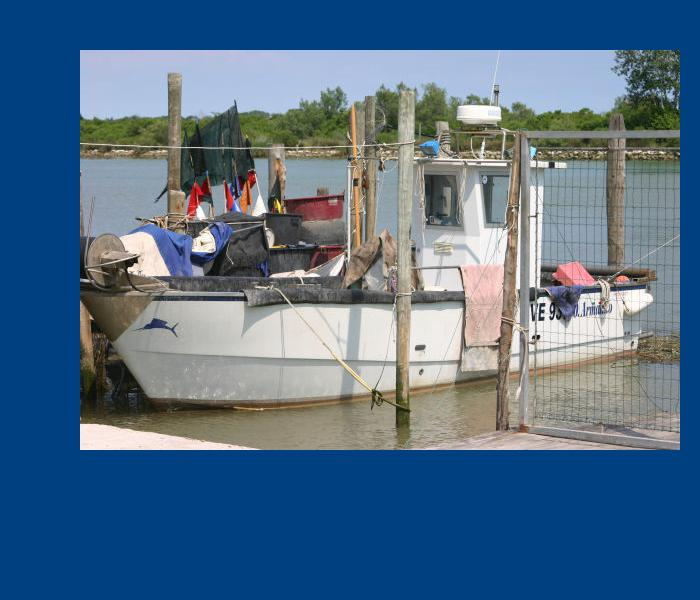The image in the centre is exactly the same size as the original. Notice that since we are adding extra pixels to the image (as a border) we need to decide what colour to make them. We have chosen a nice blue.

The image above takes the 600x400 pixel image, and pads it out to 700x600 pixels, with the image placed off-centre within the borders. Here are the measurements: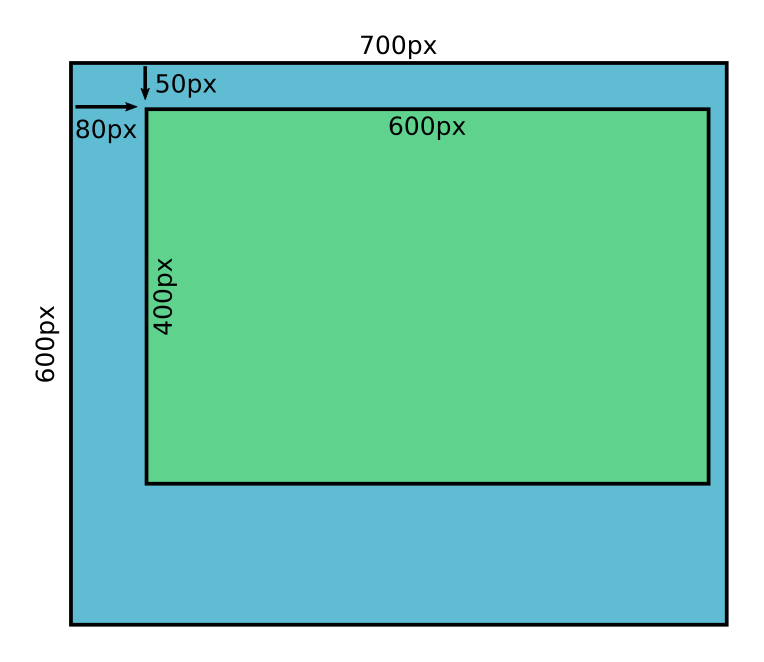The basic approach is as follows:

• Create a new array of the final image size, filled with the border colour.
• Copy the original array into a region of the new array, using numpt slicing.

Here is the code:

```img_in = Image.open('boat.jpg')
array = np.array(img_in)

padded_array = np.empty([600, 700, 3], dtype=np.uint8)
padded_array[:, :] = np.array([0, 64, 128])

```

We create `padded_array` as an empty array of the required size. We then fill the array with colour `[0, 64, 128]`, a bright blue.

Next, we copy the original `array` into a slice `[50:450, 80:680]` of the output array. Notice that the slice dimensions are exactly equal to the original image dimensions.

This code can be found as padded-image.py on github.

NumPy also has a `pad` function that can be used to pad an image, like this:

```padded_array = np.pad(array, ((50, 150), (80, 20), (0, 0)))
```

The padding is specified by the sequence:

```((50, 150), (80, 20), (0, 0))
```

This is a tuple of 3 tuples:

• `(50, 150)` specifies that the first axis (the image rows) should be padded by 50 at the start, 150 at the end. This adds a 50 pixel margin at the top of the image and a 150 pixel margin at the bottom of the image.
• `(80, 20)` specifies that the first axis (the image columns) should be padded by 80 at the start, 20 at the end. This adds an 80 pixel margin at the left of the image and a 20 pixel margin at the right of the image.
• `(0, 0)` specifies that no padding should be used on the third axis. The third axis, as we know, has a length of 3 and represents the red, green and blue values for each pixel. We don't want to pad that axis because we don't want to change the colours of the pixels in any way.

The `pad` function does the padding in a single line of code, whereas the previous method took 3 lines of code. But arguably the code is a bit more complex. The main disadvantage is that `pad` will set the pad colour to black by default: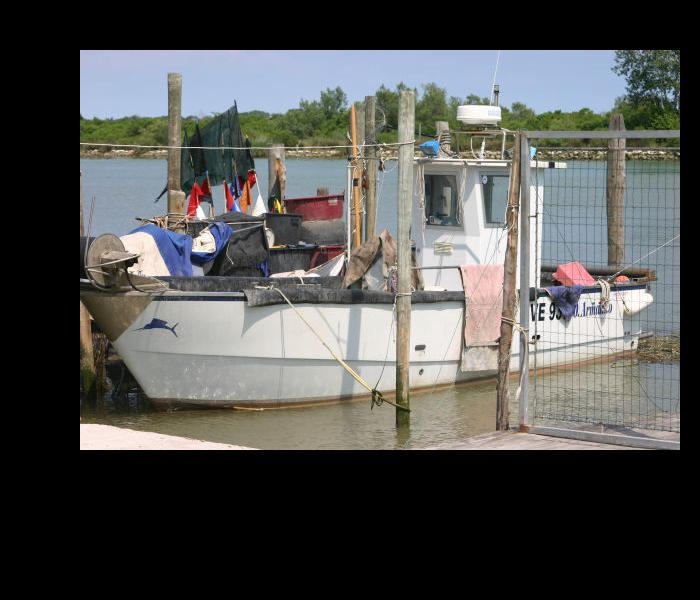You can set the value of the padding elements like this:

```padded_array = np.pad(array, ((50, 150), (80, 20), (0, 0)), constant_values=(128,))
```

This sets every added element to the value 128. However, this will set the R, G and B values of each new pixel to the same value, so you can only fill the background with a shade of grey. If you want a coloured background you should use the origtinal method, above.

However, the `pad` function also provides a `mode` parameter:

```padded_array = np.pad(array, ((50, 150), (80, 20), (0, 0)), mode='wrap')
```

Setting the `mode` to `wrap` fills the padding area with a copy of the original image, rather than black: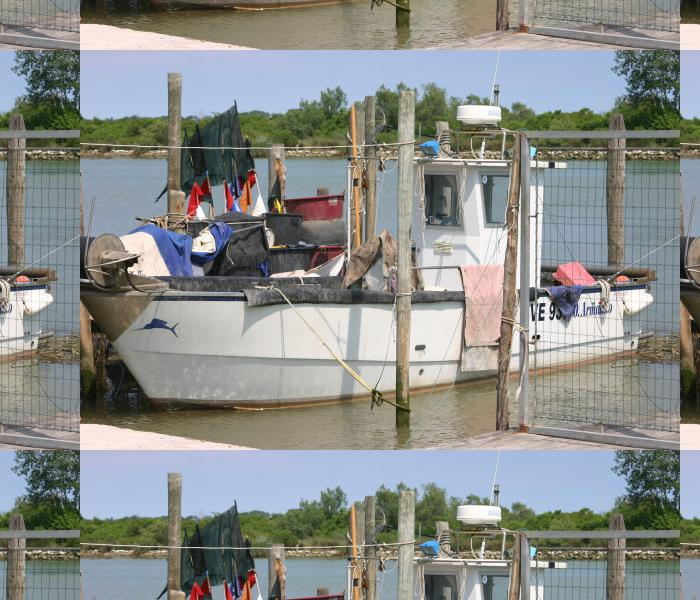This effectively tiles the original image across the padding area. You can also use `reflect`, which does a similar thing except it flips the tiles in the padded image. There are various other modes to try.

## Flipping images

We can flip an image horizontally or vertically.

Horizontal flipping, also called left-to-right flipping creates a mirror image of the original, like this:One way to do this would be to use negative indexing in NumPy, something like this:

```flipped_array = array[:, ::-1]
```

Remember that `::-1` creates a full slice but with a step of -1, in other words it reverses the array onthat axis. So the code above will reverse the order of the rows in the image, resulting in a horzontal flip.

However, NumPy has a function `fliplr` that flips an array on its second axis, so we will use that instead:

```img_in = Image.open('boat.jpg')
array = np.array(img_in)

flipped_array = np.fliplr(array)

img_out = Image.fromarray(flipped_array)
img_out.save('fliph-boat.jpg')
```

We can also flip the image top to bottom, like this:The code is identical to the previoys code, except that we use the `flipud` function (flip up/down).

This code can be found as fliph-image.py and fliph-image.py on github.

Visit the PythonInformer Discussion Forum for numeric Python.

If you found this article useful, you might be interested in the book NumPy Recipes or other books by the same author.

#### Popular tags

2d arrays abstract data type alignment and animation arc array arrays bezier curve built-in function callable object circle classes close closure cmyk colour comparison operator comprehension context context manager conversion creational pattern data types design pattern device space dictionary drawing duck typing efficiency else encryption enumerate fill filter font font style for loop function function composition function plot functools game development generativepy tutorial generator geometry gif gradient greyscale higher order function hsl html image image processing imagesurface immutable object index inner function input installing iter iterable iterator itertools l system lambda function len line linspace list list comprehension logical operator lru_cache magic method mandelbrot mandelbrot set map monad mutability named parameter numeric python numpy object open operator optional parameter or partial application path polygon positional parameter print pure function pycairo radial gradient range recipes rectangle recursion reduce rgb rotation scaling sector segment sequence singleton slice slicing sound spirograph sprite square str stream string stroke subpath symmetric encryption template text text metrics tinkerbell fractal transform translation transparency tuple turtle unpacking user space vectorisation webserver website while loop zip